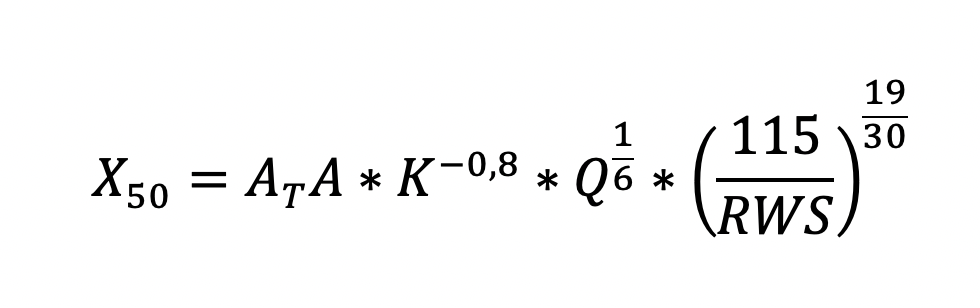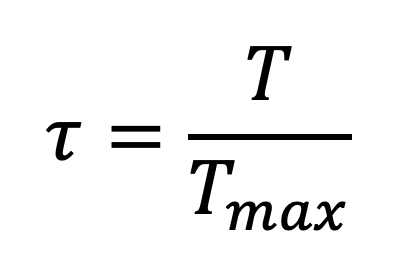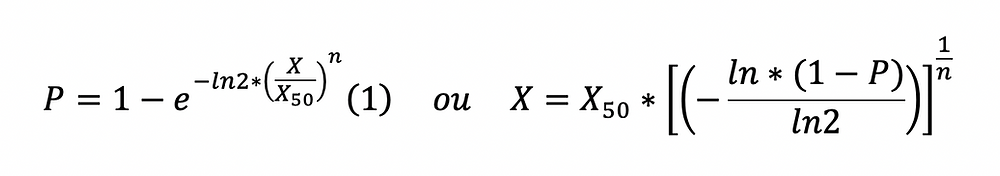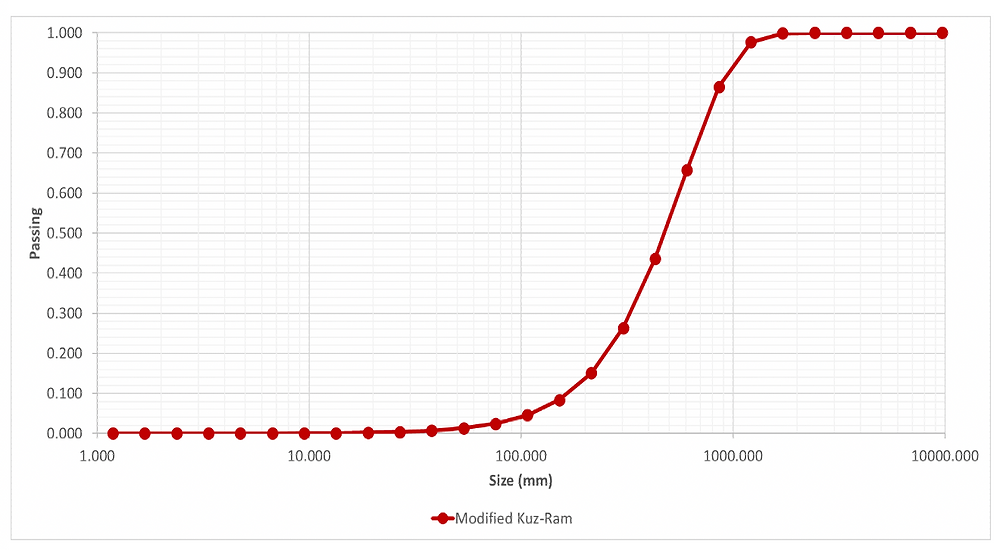Buscar

# Prediction of rock fragmentation: Kuz-Ram modify model (2005)

Today we will start with something simple from the point of view of mathematical implementation, which is the Kuz-Ram fragmentation forecast model proposed by Cunningham, in which there are three versions available (1983, 1987 and 2005). In this article we will address the most recent one, that of 2005. Despite the model that will be presented being 15 years old, it is still one of the most used worldwide. In addition to those proposed by the author, there are others such as Crush Zone Model CZM, Kuznetsov-Cunningham-Ouchterlony KCO, Multiple Blasthole Fragmentation MBF and xP-frag.

In this empirical model, Cunningham (2005) brought improvements in relation to the previous models, which are:

· Inclusion of the effect of the delay times between holes and the impact of the dispersion time;

· Change in the formula for estimating the rock factor;

· Change in the formula for estimating the uniformity index.

The Kuz-Ram model works around three expressions, the Kuznetsov equation, the uniformity index and the Rosin-Rammler equation (used to construct the accumulated curve of the fragmented material).

1 Kuznetsov equationBeing:

X50 : Sieve opening where 50% of the fragmented material is passed (cm);

At : Delay factor between holes;

A : The rock factor corresponds to the degree of difficulty of fragmentation of the rock mass, with intervals between 0.8 and 22.

K : Specific explosive consumption (kg/m³);

Q : Mass corresponding to the amount of explosive per hole (kg);

RWS : Mass relative energy of the explosive compared to pure ANFO.

1.1 Delay factor between holesFigure 1 - Tentative algorithm for the effect of interhole delay on mean fragmentation.

Based on the studies by Bergman et al (1974) in which it was sought to understand how the delay times influenced the average fragment, the authors noted that there were optimal relief times from 3 ms/m. The studies were carried out on granites with P wave speed in the rock mass, Cp, of 5,200 m / s. In order to use the delay factor between holes, Cunninghamm (2005) makes a parameterization according to the seismic speed of the studies by Bergman et al (1974), and also makes an approximation for the engineers/technicians who use the Kuz-Ram model could use the seismic velocity of the rock at the study site to predict fragmentation.

The expression is given by:Being:

Tmax : Delay timing for maximum fragmentation (ms);

At : P wave speed (km/s);

B : Burden (m).

Once the curve presented by Bergman et al (1974) is normalized, we can then define the delay factor between holes, determined as follows:In which:T: Delay time between holes (ms).

1.2 Rock factor

The rock factor is a dimensionless index that can be obtained in the classification of rock masses proposed by Lilly (1986 and 1992). It is estimated by three parameters, and quantified as follows:Cunningham (2005) presents a table with each of the parameters, being possible to obtain them from the weights that correspond to the description of the rock mass.

Table 1 - Rock characterisation: factor ABeing:

RMD: Rock mass description;

JF: Joint Factor;

RDI: Rock density index;

HF: Hardness factor.

E: Young's modulus (GPa);

RD: Density of the rock;

UCS: Uniaxial compression strength (MPa);

2 Uniformity index equationBeing:

n : The uniformity index is between 0.7 and 2.2 according to Jimeno et al (2017). The higher this value, the more uniform the fragmented material will be. And how much less, indicates significant amounts in the generation of fine and coarse materials.

Rs : Scatter ratio (uniformity will react directly to the initiation system used, electronic or non-electric);

D: Diameter (mm);

S: Spacing (m);

W: drilling error (m);

L: Length of the explosive charge (m).

2.1 Scatter ratio

“It is self-evident that, if timing influences the fragmentation in blasting, then timing scatter will affect the uniformity of blasting fragmentation. This is why there has to be adjustment both for the delay used and the scatter”.

Based on the statement made by Winzer et al (1979) about his studies of the influence of timing processes and their dispersions, Cunninghamm (2005) noted the importance of these factors and proposed the following formula for the correction of the uniformity index:Being:

σt: Standard deviation of initiation system (ms).

3 Rosin-Rammler equationBeing:

P: Represents the percentage of material passing through a “X” size sieve (%).

X: Mesh size corresponds to the sieve (mm).Figure 2 - Particle size curve generated by the Kuz-Ram model (2005).

After determining the average size, , and the uniformity index, it is possible to create the particle size distribution curve. The first formula (1) is used to determine the percentage of material passing through a given size class inserted in the variable "X". The second formula (2), on the other hand, is more used, because instead of referring us to the passing percentage of a certain class of fragments, it will estimate the size of the fragment based on the percentage entered by the user.

4 Conclusion

This is one of the models among which our team of engineers from the engineering department of O-Pitblast, the new consulting concept of our brand, O-pit Engineering, uses for the optimization and prediction of fragmentation in open-pit mines and quarries of our customers. The use of these methods and models has been of great relevance for a broad and effective solution for the resolution of adversities in the day-to-day activities of mining around the world. If your company has problems with fragmentation, contact us at engineering@o-pitblast.com, we will be pleased to hear a little about your business and solve possible problems.

Author:

Auã Kiahla

O-Pitblast ®

128 visualizações0 comentário

Ver tudo